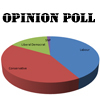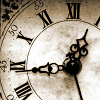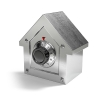Search by Topic

Resources tagged with Mixed fractions, decimals and percentages similar to Mathland Election:

Filter by: Content type:
Age range:
Challenge level:

There are 4 results

Broad Topics > Fractions, Decimals, Percentages, Ratio and Proportion > Mixed fractions, decimals and percentagesMathland Election

Age 11 to 14 Challenge Level:

A political commentator summed up an election result. Given that there were just four candidates and that the figures quoted were exact find the number of votes polled for each candidate.As Easy as 1,2,3

Age 11 to 14 Challenge Level:

When I type a sequence of letters my calculator gives the product of all the numbers in the corresponding memories. What numbers should I store so that when I type 'ONE' it returns 1, and when I type. . . .John's Train Is on Time

Age 11 to 14 Challenge Level:

A train leaves on time. After it has gone 8 miles (at 33mph) the driver looks at his watch and sees that the hour hand is exactly over the minute hand. When did the train leave the station?A Change in Code

Age 14 to 16 Challenge Level:

There are two sets of numbers. The second is the result of the first after an increase by a constant percentage. How can you find that percentage if one set of numbers is in code?